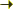Bilbao Crystallographic ServerSYMMETRY OPERATION Help

## Geometric Interpretation of Matrix Column Representation of Symmetry Operation

 Symmetry Operation
 This program calculates the geometric interpretation of matrix column representation of symmetry operation for a given crystal system or space group. Input: i) The crystal system or the space group number. ii) The matrix column representation of symmetry operation. The program is able to calculate the geometrical interpretation refer either to the standard/default setting of the chosen space group or to the so-called ITA Settings. If you want to work on a non conventional setting click on Non conventional setting, this will show you a form where you have to introduce the transformation matrix relating the conventional setting of the group you have chosen with the non conventional one you are interested in. Output: We obtain the geometric interpretation of the symmetry operation according to the International Tables for Crystallography, Vol. A. criteria and in Seitz notation.
 Introduce the crystal system triclinic monoclinic orthorhombic tetragonal trigonal hexagonal cubic Or enter the sequential number of group as given in the International Tables for Crystallography, Vol. A

Introduce the matrix-column representation of the symmetry operationin:

• Coordinate triplets
• Or in matrix form

Rotational part

Translational part